# 基于Datalore开发机器学习项目的体验+关注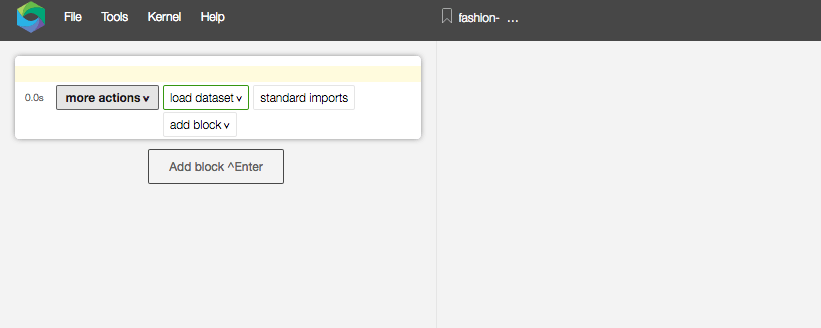workbook是左右双栏，左边是源代码，右边显示结果。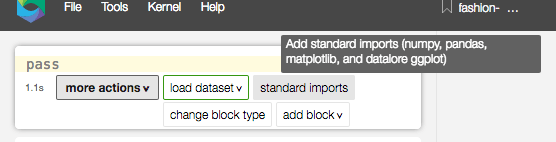import tensorflow as tf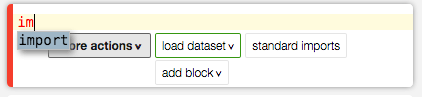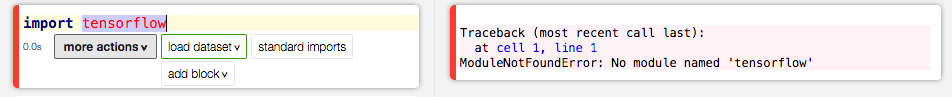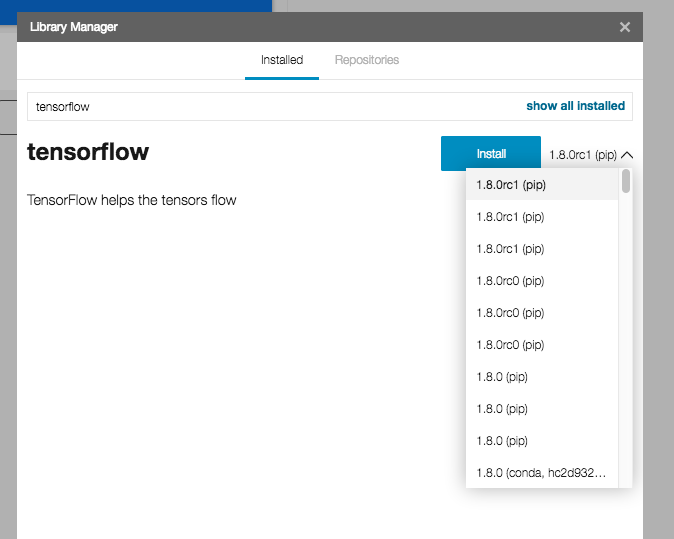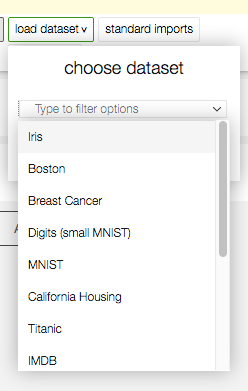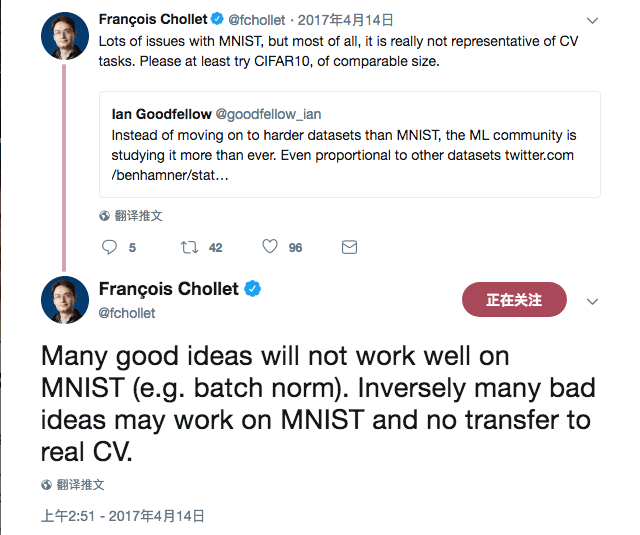“花书”作者Ian Goodfellow、Keras作者François Chollet一起炮轰MNIST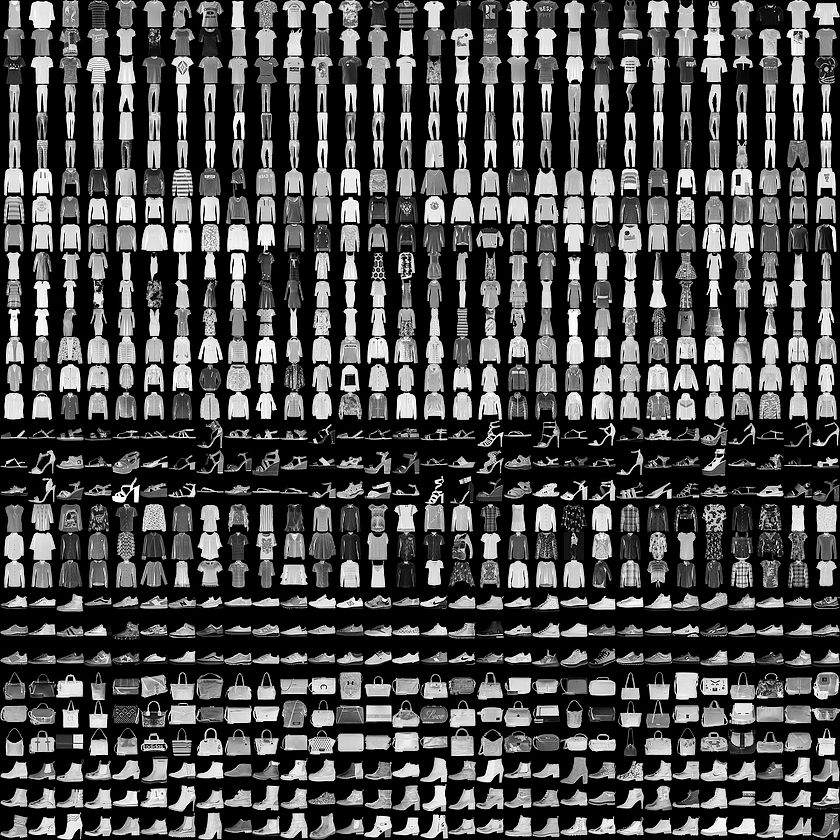TensorFlow的API写起来还是有点啰嗦，所以我决定使用Keras。而且，Keras的datasets模块自带获取和加载Fashion-MNIST的方法。

from keras.datasets import fashion_mnist

(x_train, y_train), (x_test, y_test) = fashion_mnist.load_data()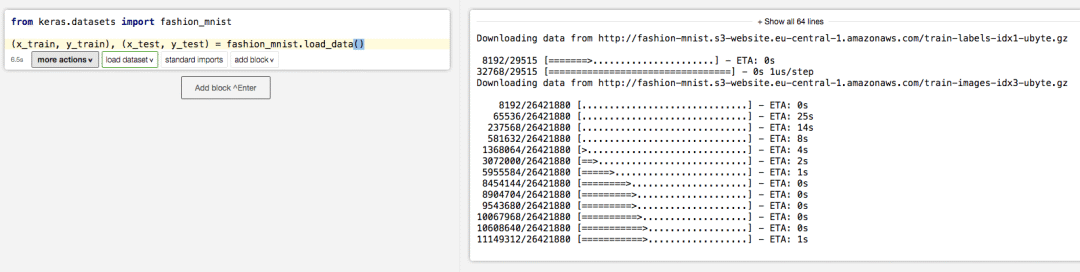x_train = x_train.astype('float32') / 255

x_train = x_train.reshape(x_train.shape, 28, 28, 1)

x_test = x_test.astype('float32') / 255

x_test = x_test.reshape(x_test.shape, 28, 28, 1)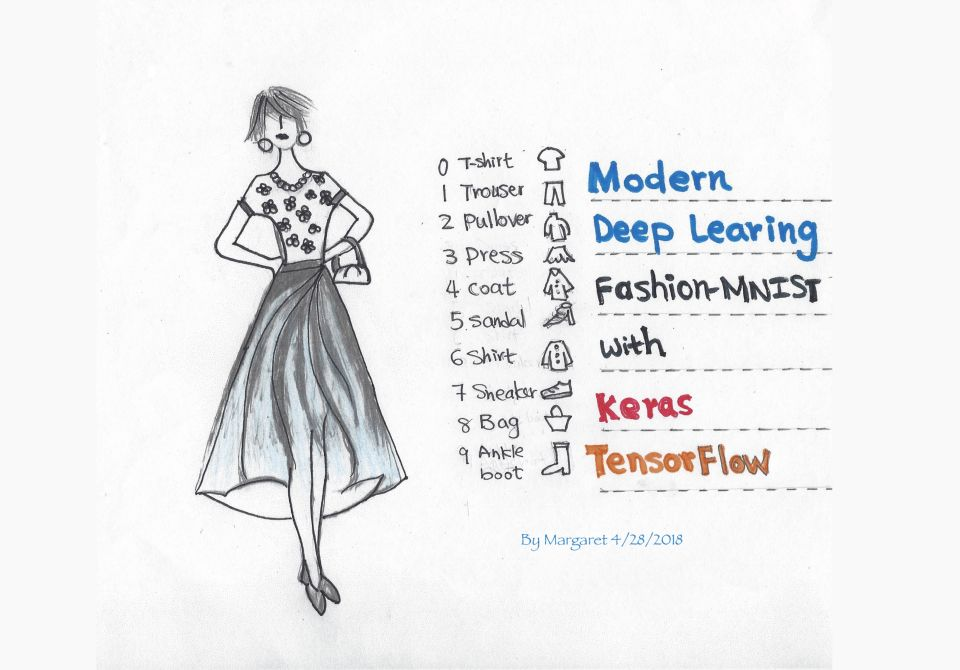y_train = keras.utils.to_categorical(y_train, 10)

y_test = keras.utils.to_categorical(y_test, 10)

model = Sequential()

model.add(Conv2D(32, kernel_size=(3, 3),

activation='relu',

input_shape=(28, 28, 1)))

model.add(Conv2D(64, (3, 3), activation='relu'))

model.add(MaxPooling2D(pool_size=(2, 2)))

model.add(Dropout(0.25))

model.add(Flatten())

model.add(Dense(128, activation='relu'))

model.add(Dropout(0.5))

model.add(Dense(10, activation='softmax'))

model.compile(loss=keras.losses.categorical_crossentropy,

optimizer=keras.optimizers.Adadelta(),

metrics=['accuracy'])

model.compile(loss=keras.losses.categorical_crossentropy,

optimizer=keras.optimizers.Adadelta(),

metrics=['accuracy'])

model.fit(x_train, y_train,

batch_size=128,

epochs=20,

verbose=1,

validation_data=(x_test, y_test))

score = model.evaluate(x_test, y_test, verbose=0)

print('测试损失', score)

print('测试精确度', score)

20个epoch后精确度超过90%，比我预料中的表现要好。

from keras.datasets import mnist

(x_train, y_train), (x_test, y_test) = mnist.load_data()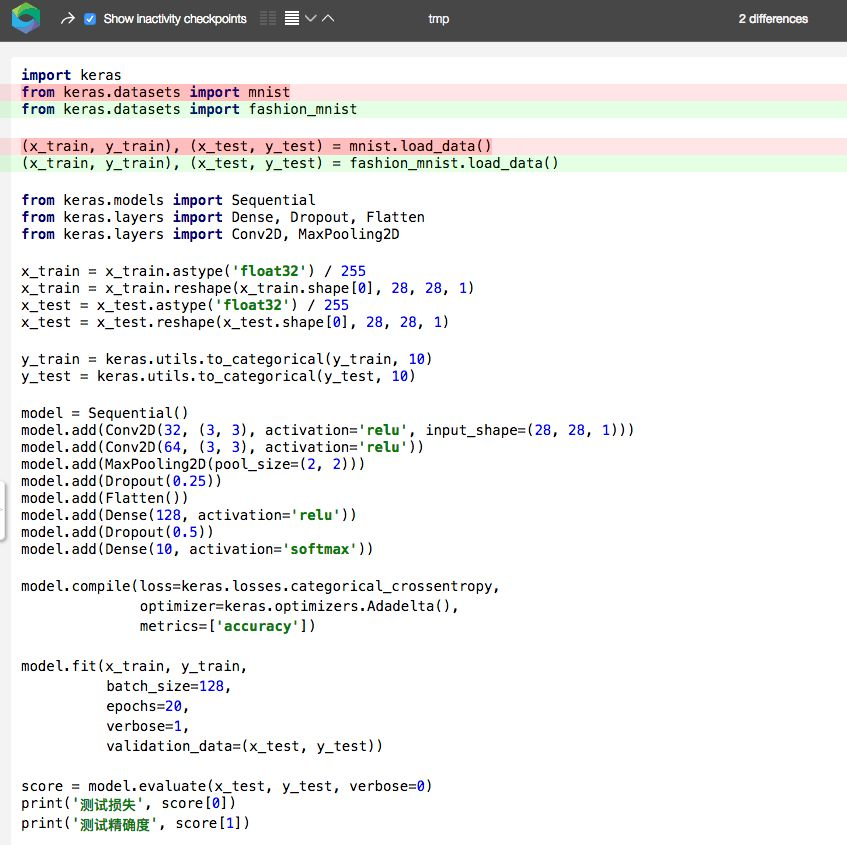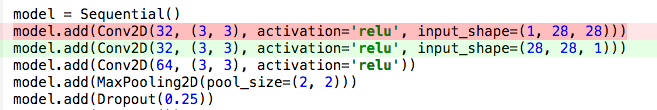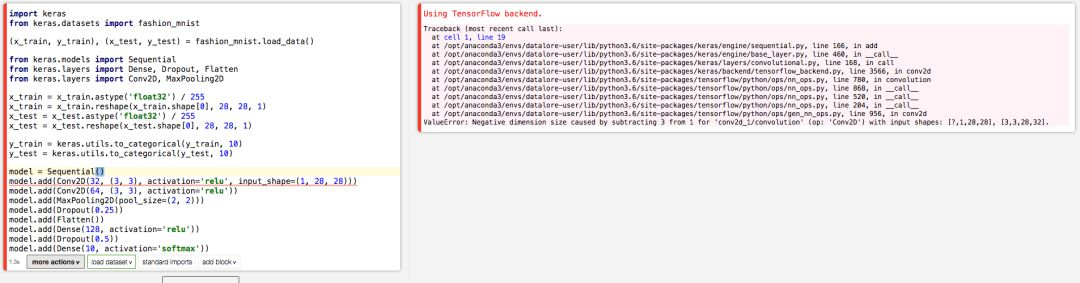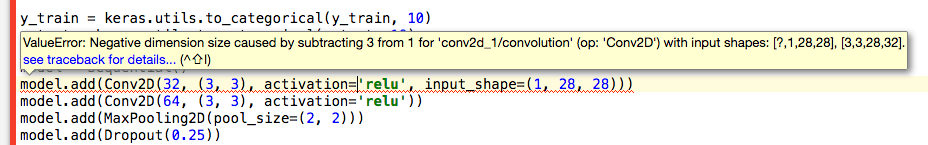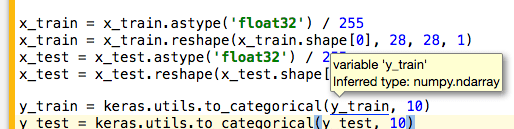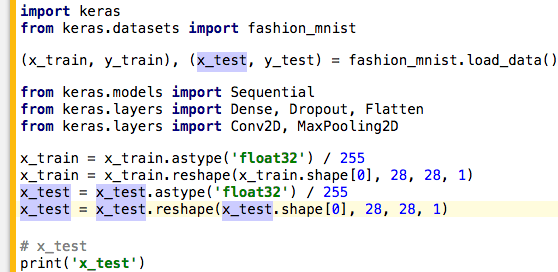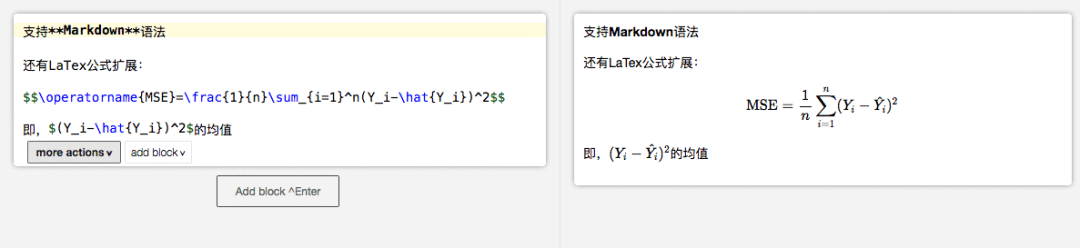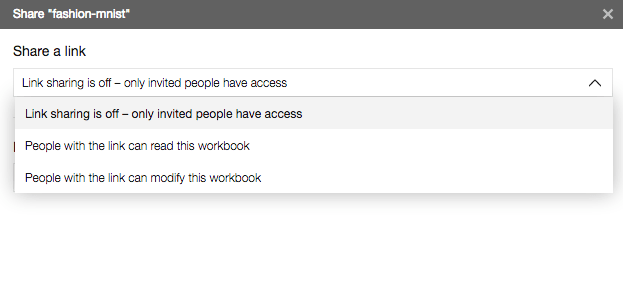C-空格 （C在mac os x上是Command键，其他平台上是ctrl键）可以触发自动补全。

C-d 重复当前行